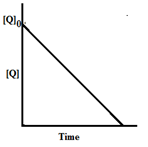×#### Thank you for registering.

One of our academic counsellors will contact you within 1 working day.

Click to Chat

1800-1023-196

+91-120-4616500

CART 0

• 0

MY CART (5)

Use Coupon: CART20 and get 20% off on all online Study Material

ITEM
DETAILS
MRP
DISCOUNT
FINAL PRICE
Total Price: Rs.

There are no items in this cart.
Continue Shopping• Complete JEE Main/Advanced Course and Test Series
• OFFERED PRICE: Rs. 15,900
• View Details

```Solved Examples on Chemical Kinetics

Question:1 )The rate of a reaction doubles when its temperature changes from 300K to 310K. Activation energy of such a reaction will be: (R = 8.314 JK–1mol–1and log 2 = 0.301)  (IIT JEE-2013)

1) 48.6 kJ mol–1

2) 58.5 kJ mol–1

3) 60.5 kJ mol–1

4) 53.6 kJ mol–1

Solution: The question is based on the effect of temperature change on rate of reaction and involves the concept of activation energy. So, Arrhenius equation should be used for solving this problem.

Using Arrhenius Equation

ln(k2/k1) = (Ea/R)(1/T1-1/T2)

We get,

2.303 log2 = -( Ea/8.314)(1/300 -1/310)

Ea = 5359.59 Jmol-1 = 53.6 kJ mol-1

Hence, the correct option is D.Question:2) In the reaction  P + Q → R + S, the time taken for 75% reaction of P is twice the time taken for 50% reaction of P. The concentration of Q varies with reaction time as shown in the figure. The overall order of the reaction is  (IIT JEE -2013)1) 2

2) 3

3) 0

4) 1

Solution:

Time required for 75% reaction of P is twice the time taken for 50% reaction, this indicates that the half life of the reaction is independent of the initial concentration and remains constant throughout the reaction.

For first order kinetics, after first half life the concentration of P decreases up to 50 %.

After 2nd half life the concentration of P once again decreases to its half value and now it reduced up to 25% of the initial concentration.

From the graph it is clear that reaction is zero order with respect to Q.

R =k1[P]1[Q]0

Overall order of the reaction = 1+0 =1.

Hence, the correct option is D.

Question:3) An organic compound undergoes first-order decomposition. The time taken for its decomposition to 1/8 and 1/10 of its initial concentration are t1/8 and t1/10 respectively. What is the value of [t1/8 ] / [t1/10 ] × 10 ?  (Take log2 = 0.3) (IIT JEE -2012)

Solution:```### Course Features

• 728 Video Lectures
• Revision Notes
• Previous Year Papers
• Mind Map
• Study Planner
• NCERT Solutions
• Discussion Forum
• Test paper with Video Solution